# Geometry 101: Beginner to Intermediate Level\$85.00
no certificate
- OR -
\$110.00
with CEU Certificate*
Start Right Now!
Taking multiple courses? Save with our platinum program.

• 15
Lessons
• 21
Exams &
Assignments
• 2,012
Students
have taken this course
• 17
Hours
average time
• 1.7
CEUs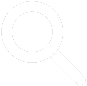## Course Description

Although we may not always recognize it, we use basic geometry skills regularly in everyday life. For instance, we consider whether a picture on the wall is parallel with the floor, or we calculate the area of a room when installing new carpeting.

Geometry is also an integral part of many other areas of study, including physics and other natural sciences. This course provides a more rigorous mathematical foundation for both practical and theoretical geometric thinking.

The course begins by considering basic figures such as points, lines, and planes, and then expands into the concepts of parallelism and perpendicularity as well as more complicated geometric figures such as polygons (triangles, quadrilaterals, and so on) and circles. The course considers mainly two-dimensional figures, but two lessons on three-dimensional geometry provide more complete coverage of the subject.

Each successive lesson in the course builds on previous lessons, culminating in lessons that teach the student how to calculate lengths, areas, and volumes of more complicated figures. In addition to its in-depth discussion of the mathematical aspects of geometry, the course also includes lessons on how to draw various figures using a compass and straightedge as well as a lesson on strategy for solving real-world geometry problems.

The course concludes by applying all that the student has learned to a brief introduction to trigonometry, thereby providing a jumping-off point for a more rigorous study of geometry as well as other areas of mathematics. By the end of the course, the student should feel comfortable tackling a range of basic to moderately difficult geometry problems.
• Completely Online
• Self-Paced
• 6 Months to Complete
• Start Anytime
• PC & Mac Compatible
• Android & iOS Friendly
• Accredited CEUs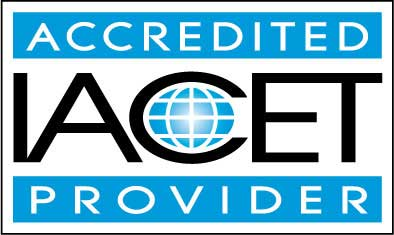## Course Lessons

Average Lesson Rating: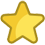#### Lesson 1 - Geometry Terms and Motivation

To begin the course, we first discuss why the study of geometry can be beneficial, both in school and work as well as in daily life. 16 Total Points
•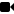Lesson 1 Video A
•Lesson 1 Video B : How to Take an Exam
•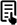Review Article: Geometry Glossary
•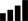Take Survey: Reasons for Taking this Course
•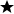Complete: Lesson 1 Assignment
•Complete: Lesson 1 Exercises
•Complete Exam: Lesson 1 Geometry Terms and Motivation

#### Lesson 2 - Geometric Reasoning and Measurement

To aid in our discussion of geometry, this lesson reviews some fundamental principles of geometric reasoning and measurement that will aid our analysis throughout the course. 10 Total Points
•Lesson 2 Video
•Complete Exam: Lesson 2 Geometric Reasoning and Measurement

#### Lesson 3 - Angles and Parallelism

In this lesson, we introduce the concept of an angle and discuss the relationships among angles formed by intersecting lines. 9 Total Points
•Lesson 3 Video
•Review Article: Types of Angles
•Complete Exam: Lesson 3 Angles and Parallelism

#### Lesson 4 - Triangles I: Properties of Triangles

This lesson introduces our first polygon: the triangle. We consider what constitutes a triangle as well as several types of triangles with special properties. 13 Total Points
•Lesson 4 Video
•Review 2 Articles: Triangle Practice Problems--Angles; Triangle Practice Problems--Area
•Review Video: Simplify Radicals
•Complete: Lesson 4 Exercises
•Complete Exam: Lesson 4 Triangles I: Properties of Triangles

#### Lesson 5 - Triangles II: Congruent Triangles

This lesson uses concepts from our introduction to triangles in the previous lesson and applies them to congruent triangles. 13 Total Points
•Lesson 5 Video
•Complete: Lesson 5 Exercises
•Complete Exam: Lesson 5 Triangles II: Congruent Triangles

#### Lesson 6 - Triangles III: Similar Triangles

This lesson concludes our focus on triangles by considering another sense in which we can understand congruence and similarity 7 Total Points
•Lesson 6 Video
•Review Article: Similar triangles
•Complete Exam: Lesson 6 Triangles III: Similar Triangles

This lesson introduces quadrilaterals, or four-sided polygons. We study the basic properties of quadrilaterals and go on to identify special quadrilaterals and to derive formulas for their areas. 10 Total Points
•Lesson 7 Video
•Review Article: Quadrilateral Overview
•Complete Exam: Lesson 7 Quadrilaterals

#### Lesson 8 - Polygons

Having studied three- and four-sided figures (polygons), we now turn to arbitrary n-sided polygons. 17 Total Points
•Lesson 8 Video
•Complete: Lesson 8 Exercises
•Complete Exam: Lesson 8 Polygons

#### Lesson 9 - Circles

The course now turns from polygons to circles. A circle is vastly different from a polygon, and it requires a different approach to understanding its properties and calculating corresponding values. 10 Total Points
•Lesson 9 Video
•Complete Exam: Lesson 9 Circles

#### Lesson 10 - Composite Figures

This lesson combines the principles of previous lessons and applies them to composite figures, which are composed of (or can be broken down into) various simpler figures. 10 Total Points
•Lesson 10 Video
•Complete Exam: Lesson 10 Composite Figures

#### Lesson 11 - Classical Construction

This lesson takes a slight break from the analytical approach to geometry and considers how to perform some simple "classical constructions" using a pencil, compass, and straightedge. 10 Total Points
•Lesson 11 Video
•Review Article: Geometrical Construction
•Complete Exam: Lesson 11 Classical Construction

#### Lesson 12 - Practical Geometry Problems

This lesson takes a break from introducing new geometry concepts and turns instead to application of what we already know to practical situations. 10 Total Points
•Lesson 12 Video
•Review Article: Geometry Word Problems
•Complete Exam: Lesson 12 Practical Geometry Problems

#### Lesson 13 - Three-Dimensional Geometry I

In this lesson, we introduce three-dimensional geometry as an extension of two-dimensional geometry. 10 Total Points
•Lesson 13 Video
•Review Article: Solid Geometry
•Complete Exam: Lesson 13 Three-Dimensional Geometry I

#### Lesson 14 - Three-Dimensional Geometry II

In this lesson, we extend our knowledge of two-dimensional geometry and what we have learned thus far about three-dimensional geometry to the analysis of simple polyhedra as well as spheres and cylinders. 10 Total Points
•Lesson 14 Video
•Complete Exam: Lesson 14 Three-Dimensional Geometry II

#### Lesson 15 - Geometry and Trigonometry

In this lesson, we introduce trigonometry, which expands on our knowledge of circles, angles, triangles, and other aspects of geometry. 53 Total Points
•Lesson 15 Video
•Take Poll: What do you think about this course?
•Take Survey: Program Evaluation Follow-up Survey (End of Course)
•Complete Exam: Lesson 15 Geometry and Trigonometry
•Complete: The Final Exam
208
Total Course Points

## Learning Outcomes

By successfully completing this course, students will be able to:
• Define basic geometry terms.
• Define geometric reasoning and measurement.
• Describe angles and parallelism.
• Describe properties of triangles.
• Describe congruent triangles.
• Describe similar triangles.
• Solve problems involving Polygons.
• Solve problems involving circles.
• Describe classical construction.
• Solve practical geometry problems.
• Describe three-dimensional geometry, and
• Demonstrate mastery of lesson content at levels of 70% or higher.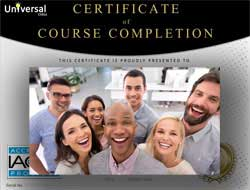• Document Your Lifelong Learning Achievements
• Earn an Official Certificate Documenting Course Hours and CEUs
• Verify Your Certificate with a Unique Serial Number OnlineCourse Title: Geometry 101: Beginner to Intermediate Level
Course Number: 9770452
Lessons Rating: 4.6 / 5 Stars (965 votes)
Languages: English - United States, Canada and other English speaking countries
Category:
Course Type: General Education (Self-Paced, Online Class)
CEU Value: 1.7 IACET CEUs (Continuing Education Units)
CE Accreditation: Universal Class, Inc. has been accredited as an Authorized Provider by the International Association for Continuing Education and Training (IACET).
Assessment Method: Lesson assignments and review exams
Course Author: Dr. Jeffrey Clark, Ph.D.
Instructor: April Cordry-Moore
Syllabus: View Syllabus
Duration: Continuous: Enroll anytime!
Course Fee: \$85.00 (no CEU Certification) || with Online CEU Certification: \$110.00

Course Only
One Course
No Certificate / No CEUs
\$85
for 6 months

Billed once
This course only
 Includes certificate X Includes CEUs X Self-paced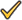Instructor supportTime to complete 6 months No. of courses 1 course
Certificate Course
One Course
Certificate & CEUs
\$110
for 6 months

Billed once
This course only
 Includes certificateIncludes CEUsSelf-pacedInstructor supportTime to complete 6 months No. of courses 1 course
Platinum Yearly
ALL COURSES
Certificates & CEUs
\$189
per year
You save 50%!
Billed once
Includes all 500+ courses
 Includes certificateIncludes CEUsSelf-pacedInstructor supportTime to complete 12 Months No. of courses 500+
Platinum 2 Years
ALL COURSES
Certificates & CEUs
\$299
for 2 years
You save \$427.00!
Billed once
Includes all 500+ courses
 Includes certificateIncludes CEUsSelf-pacedInstructor supportTime to complete 24 Months No. of courses 500+

## Student Testimonials

• "This was a great course. I learned a lot about Geometry and am excited to use what I learned here in my high school. It was definitely better than some of the other Universal courses I took because this instructor, Mr. Clark, was more organized and thorough with his lessons, and it was easier to understand him than other university's instructors." -- Hannah Z.
• "He was an excellent teacher...the lessons were very clear and easy to understand. I also like the 15 lesson layout." -- Rayna K.
• "Course was a bit difficult for me, instructor was available and helped me when I needed help!" -- Marcus M.